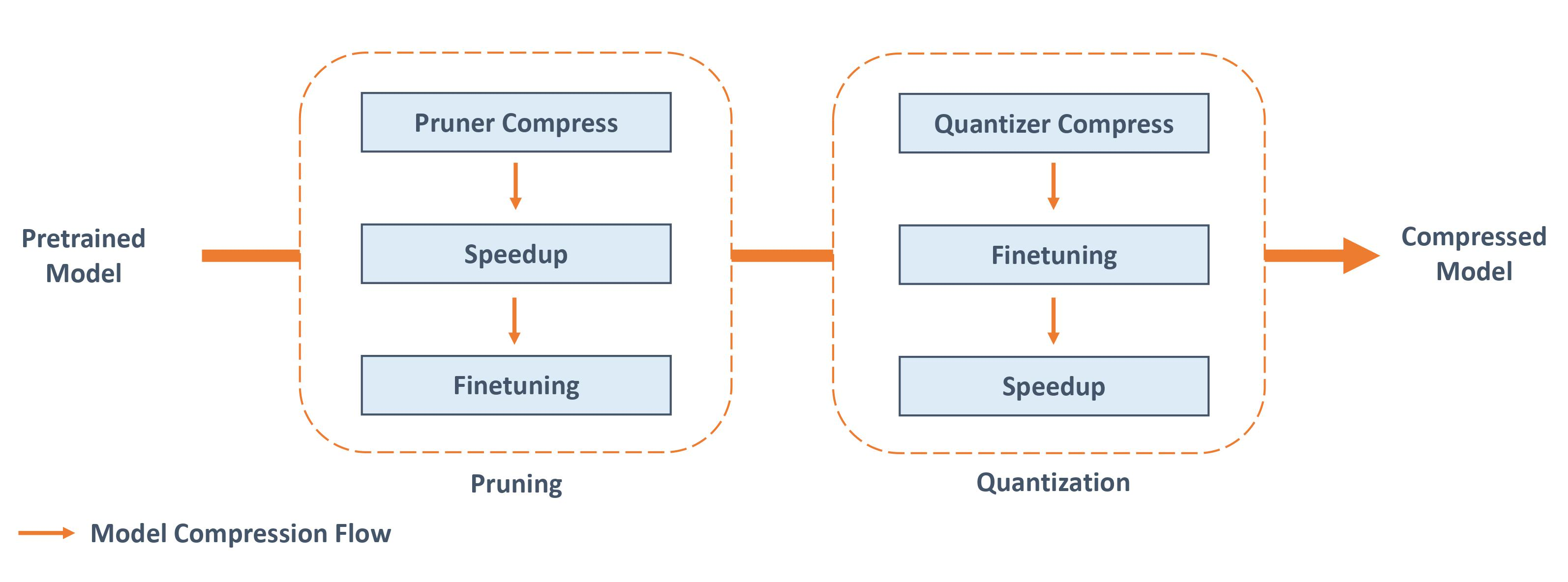# Model Compression with NNI¶

As larger neural networks with more layers and nodes are considered, reducing their storage and computational cost becomes critical, especially for some real-time applications. Model compression can be used to address this problem.

NNI provides a model compression toolkit to help user compress and speed up their model with state-of-the-art compression algorithms and strategies. There are several core features supported by NNI model compression:

• Support many popular pruning and quantization algorithms.

• Automate model pruning and quantization process with state-of-the-art strategies and NNI’s auto tuning power.

• Speed up a compressed model to make it have lower inference latency and also make it become smaller.

• Provide friendly and easy-to-use compression utilities for users to dive into the compression process and results.

• Concise interface for users to customize their own compression algorithms.

## Compression Pipeline¶The overall compression pipeline in NNI. For compressing a pretrained model, pruning and quantization can be used alone or in combination.

Note

Since NNI compression algorithms are not meant to compress model while NNI speedup tool can truly compress model and reduce latency. To obtain a truly compact model, users should conduct model speedup. The interface and APIs are unified for both PyTorch and TensorFlow, currently only PyTorch version has been supported, TensorFlow version will be supported in future.

## Supported Algorithms¶

The algorithms include pruning algorithms and quantization algorithms.

### Pruning Algorithms¶

Pruning algorithms compress the original network by removing redundant weights or channels of layers, which can reduce model complexity and mitigate the over-fitting issue.

Name

Brief Introduction of Algorithm

Level Pruner

Pruning the specified ratio on each weight based on absolute values of weights

AGP Pruner

Automated gradual pruning (To prune, or not to prune: exploring the efficacy of pruning for model compression) Reference Paper

Lottery Ticket Pruner

The pruning process used by “The Lottery Ticket Hypothesis: Finding Sparse, Trainable Neural Networks”. It prunes a model iteratively. Reference Paper

FPGM Pruner

Filter Pruning via Geometric Median for Deep Convolutional Neural Networks Acceleration Reference Paper

L1Filter Pruner

Pruning filters with the smallest L1 norm of weights in convolution layers (Pruning Filters for Efficient Convnets) Reference Paper

L2Filter Pruner

Pruning filters with the smallest L2 norm of weights in convolution layers

ActivationAPoZRankFilterPruner

Pruning filters based on the metric APoZ (average percentage of zeros) which measures the percentage of zeros in activations of (convolutional) layers. Reference Paper

ActivationMeanRankFilterPruner

Pruning filters based on the metric that calculates the smallest mean value of output activations

Slim Pruner

Pruning channels in convolution layers by pruning scaling factors in BN layers(Learning Efficient Convolutional Networks through Network Slimming) Reference Paper

TaylorFO Pruner

Pruning filters based on the first order taylor expansion on weights(Importance Estimation for Neural Network Pruning) Reference Paper

Pruning based on ADMM optimization technique Reference Paper

Automatically simplify a pretrained network to meet the resource budget by iterative pruning Reference Paper

SimulatedAnnealing Pruner

Automatic pruning with a guided heuristic search method, Simulated Annealing algorithm Reference Paper

AutoCompress Pruner

Automatic pruning by iteratively call SimulatedAnnealing Pruner and ADMM Pruner Reference Paper

AMC Pruner

AMC: AutoML for Model Compression and Acceleration on Mobile Devices Reference Paper

Pruning attention heads from transformer models either in one shot or iteratively.

You can refer to this benchmark for the performance of these pruners on some benchmark problems.

### Quantization Algorithms¶

Quantization algorithms compress the original network by reducing the number of bits required to represent weights or activations, which can reduce the computations and the inference time.

Name

Brief Introduction of Algorithm

Naive Quantizer

Quantize weights to default 8 bits

QAT Quantizer

Quantization and Training of Neural Networks for Efficient Integer-Arithmetic-Only Inference. Reference Paper

DoReFa Quantizer

DoReFa-Net: Training Low Bitwidth Convolutional Neural Networks with Low Bitwidth Gradients. Reference Paper

BNN Quantizer

Binarized Neural Networks: Training Deep Neural Networks with Weights and Activations Constrained to +1 or -1. Reference Paper

LSQ Quantizer

Learned step size quantization. Reference Paper

Observer Quantizer

Post training quantizaiton. Collect quantization information during calibration with observers.

## Model Speedup¶

The final goal of model compression is to reduce inference latency and model size. However, existing model compression algorithms mainly use simulation to check the performance (e.g., accuracy) of compressed model, for example, using masks for pruning algorithms, and storing quantized values still in float32 for quantization algorithms. Given the output masks and quantization bits produced by those algorithms, NNI can really speed up the model. The detailed tutorial of Masked Model Speedup can be found here, The detailed tutorial of Mixed Precision Quantization Model Speedup can be found here.

## Compression Utilities¶

Compression utilities include some useful tools for users to understand and analyze the model they want to compress. For example, users could check sensitivity of each layer to pruning. Users could easily calculate the FLOPs and parameter size of a model. Please refer to here for a complete list of compression utilities.